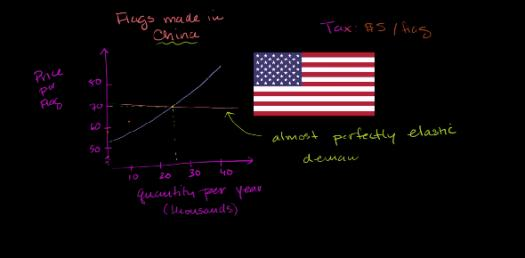# Elasticity And Per-unit Tax

20 Questions | Attempts: 364
ShareSettingsYou will need the graph from class to answer some of the following questions. If the question asks for a number (dollar or quantity, just input the number without any dollar signs--> ex: \$30 would be inputed 30)

• 1.
Using graph 1, a \$20 per-unit tax is placed on the product. What is the quantity sold with the per-unit tax?
• 2.
What is the price charged in the market after the per-unit tax?
• 3.
What is the dollar value of the tax revenue the government collects?
• 4.
Using graph 1, what is the dollar value of the total revenue the firm receives after it remits the taxes to the government?
• 5.
Using graph 1, what is the dollar value of consumer surplus after the \$20 per unit tax?
• 6.
Using graph 1, what is the dollar value of producer surplus after the \$20 per unit tax?
• 7.
Using graph 1, what is the dollar value of the deadweight loss after the \$20 per unit tax?
• 8.
Using graph 1, what is the dollar value of total revenue the firm receives after it remits the taxes to the government with a \$40 per unit tax?
• 9.
The elasticity of demand measures the responsiveness of: A. Demand to price changeB. Quantity demanded to price changeC. Shifts in demand when price changesD. Changes in total expenditures when total revenues changeE. Changes in total revenues when total expenditures change
• A.

A

• B.

B

• C.

C

• D.

D

• 10.
If a good is considered a necessity, which of the following is most likely to occur?A The demand will be more elasticB The demand curve will be flatterC The quantity demanded is likely to be more responsive to price changesD The quantity demanded is likely to be less responsive to price changesE A price increase is likely to lead to less total revenue
• A.

A

• B.

B

• C.

C

• D.

D

• E.

E

• 11.
If scientists discover alternative ways of powering automobiles and markets are created for these alternative fuels, the demand for gasoline is going to become:more elasticA less elasticB more costlyC indeterminateD more plentiful
• A.

A

• B.

B

• C.

C

• D.

D

• 12.
Assuming that good A and good B currently sell at the same price.  Good A has a more vertical demand curve than good B.  Both goods A and B experience a 10% incrase in price, then:A Good A will have a greater decrease in total revenue than good BB Good B will experience a greater increase in total expenditures than good AC Good A will have a greater decrease in total expenditures than good BD We can not determine what will happen to total revenues for good A compared to good BE Good A will experience a greater increase in total revenue than good B
• A.

A

• B.

B

• C.

C

• D.

D

• E.

E

• 13.
If the price of chocolate-covered peanuts decreases from \$1.10 to \$0.90 and the quantity demanded increases from 190 bags to 210 bags, this indicates that, if other things are unchanged, the price elasticity of demand using the midpoint method is:
• A.

0

• B.

.5

• C.

1

• D.

2

• 14.
If the price of chocolate-covered peanuts decreases from \$1.10 to \$0.90 and the quantity demanded increases from 180 bags to 220 bags, this indicates that, if other things are unchanged, the price elasticity of demand using the midpoint method is:
• A.

0

• B.

.5

• C.

1

• D.

2

• 15.
If the price of chocolate-covered peanuts decreases from \$1.10 to \$0.90 and the quantity demanded does not change, this indicates that, if other things are unchanged, the price elasticity of demand using the midpoint method is:
• A.

0

• B.

.5

• C.

1

• D.

2

• 16.
The price of gasoline rises 5% and the quantity of gasoline purchased falls 1%. The price elasticity of demand is equal to ________ and demand is described as ________.  (format: answer,answer)
• 17.
The price of Good X is \$5 and at that price consumers demand 12 units. If the price rises to \$7, consumers will decrease consumption to 4 units. Use the midpoint formula to calculate the price elasticity of demand for Good X.
• A.

1/3

• B.

3

• C.

1/6

• D.

6

• 18.
A local restaurant has estimated that the price elasticity of demand for meals is equal to 2. If the restaurant increases menu prices by 5%, they can expect the number of customers to decrease by ________and total revenue to ________.
• A.

10%; increase

• B.

5%; stay constant

• C.

10%; fall

• D.

2.5%; fall

• 19.
If the price of burritos increases from \$4 to \$6, and customers decrease their consumption from 20 to 10 burritos, what is the price elasticity of demand, using the midpoint method?
• A.

5/3

• B.

2/3

• C.

3/1

• D.

2/1

• 20.
Which of the following best describes price elasticity of demand?
• A.

Price elasticity of demand measures the responsiveness of the change in the quantity demanded to a change in price.

• B.

Price elasticity of demand measures the change in price versus a change in quantity demanded.

• C.

Price elasticity of demand measures the responsiveness of the change in slope of the demand curve to a change in price.

• D.

Price elasticity of demand measures the change in slope of the demand curve versus a change in quantity demanded.

## Related TopicsBack to top
×

Wait!
Here's an interesting quiz for you.